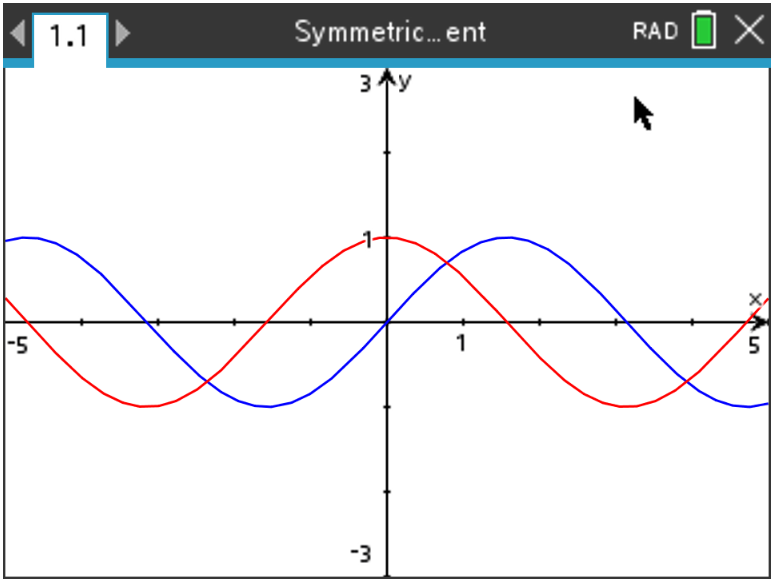# Activities

•• ##### Subject Area

• Math: Calculus: Derivatives

9-12

50 Minutes

• ##### Device
•TI-Nspire™ CX/CX II
•TI-Nspire™ CX CAS/CX II CAS
• ##### Software

TI-Nspire™ CX
TI-Nspire™ CX CAS

5.0

## Investigating the Derivatives of Some Common Functions#### Activity Overview

In this activity, students will investigate the derivatives of sine, cosine, natural log, and natural exponential functions by examining the symmetric difference quotient at many points using the table capabilities of the graphing handheld.

#### Objectives

Students will:

• Develop the idea of the derivative as a function.
• Gather evidence toward some common derivative formulas.
• Use numerical and graphical investigations to form conjectures.

#### Vocabulary

• derivatives
• symmetric difference quotient

#### About the Lesson

One of the many ways in which you can think of a derivative is as a function that uses x as an input and returns the slope of the line tangent to f at x. The derivative of a function is often another function with a formula that can be used and applied. In this activity, you will investigate the derivatives of some common functions by approximating the instantaneous rate of change (using the symmetric difference quotient) at many inputs. You will also use the table and graphing capabilities of your graphing handheld.